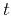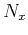Seismic wave extrapolation using lowrank symbol approximationNext: LOWRANK APPROXIMATION Up: Fomel, Ying, & Song: Previous: INTRODUCTION

# WAVE EXTRAPOLATION

Letbe the seismic wavefield at locationand time. The wavefield at the next time stepcan be approximated by the following mixed-domain operator (Wards et al., 2008)(1)

whereis the spatial Fourier transform of(2)

whereis the spatial wavenumber. To define the phase function, which appears in equation (1), one can substitute approximation (1) into the wave equation and extract the geometrical (high-frequency) asymptotic of it. In case of seismic wave propagation, this leads to the eikonal-like equation(3)

whereis the phase velocity, and the choice of the sign corresponds, in the case of a point source, to expanding or contracting waves. In the isotropic case,does not depend on. The initial condition for equation (3) is(4)

which turns equation (1) into the simple inverse Fourier transform operation.

Assuming small stepsin equation (1), one can build successive approximations for the phase functionby expanding it into a Taylor series. In particular, let us represent the phase function as(5)

Correspondingly,(6)

Substituting expansions (5) and (6) into equation (3) and separating terms with different powers of, we find that(7)(8)

When either the velocity gradientor the time stepare small, the Taylor expansion (5) can be reduced to only two terms, which in turn reduces equation (1) to the familiar expression (Etgen and Brandsberg-Dahl, 2009)(9)

or(10)

In rough velocity models, where the gradientdoes not exist, one can attempt to solve the eikonal equation 3 numerically or to apply approximations other than the Taylor expansion (5). In the examples of this paper, we used only theterm.

Note that the approximations that we use, starting from equation (1), are focused primarily on the phase of wave propagation. As such, they are appropriate for seismic migration but not necessarily for accurate seismic modeling, which may require taking account of amplitude effects caused by variable density and other elastic phenomena.

The computational cost for a straightforward application of equation (1) is, whereis the total size of the three-dimensionalgrid. Even for modest-size problems, this cost is prohibitively expensive. In the next section, we describe an algorithm that reduces the cost to, whereis a small number.Seismic wave extrapolation using lowrank symbol approximationNext: LOWRANK APPROXIMATION Up: Fomel, Ying, & Song: Previous: INTRODUCTION

2013-08-31